# NCERT Solutions for Class 9 Science Chapter 11 – Work and Energy

NCERT Solutions for Class 9 Science Chapter 11 – Work and Energy, contains solutions to various questions in Exercises for Chapter 11. Work and Energy Class 9 NCERT Solutions have been explained in a simple and easy to understand manner. We are providing all subjects which can be accessed by clicking here.

## Download NCERT Solutions for Class 9 Science Chapter 11 – Work and Energy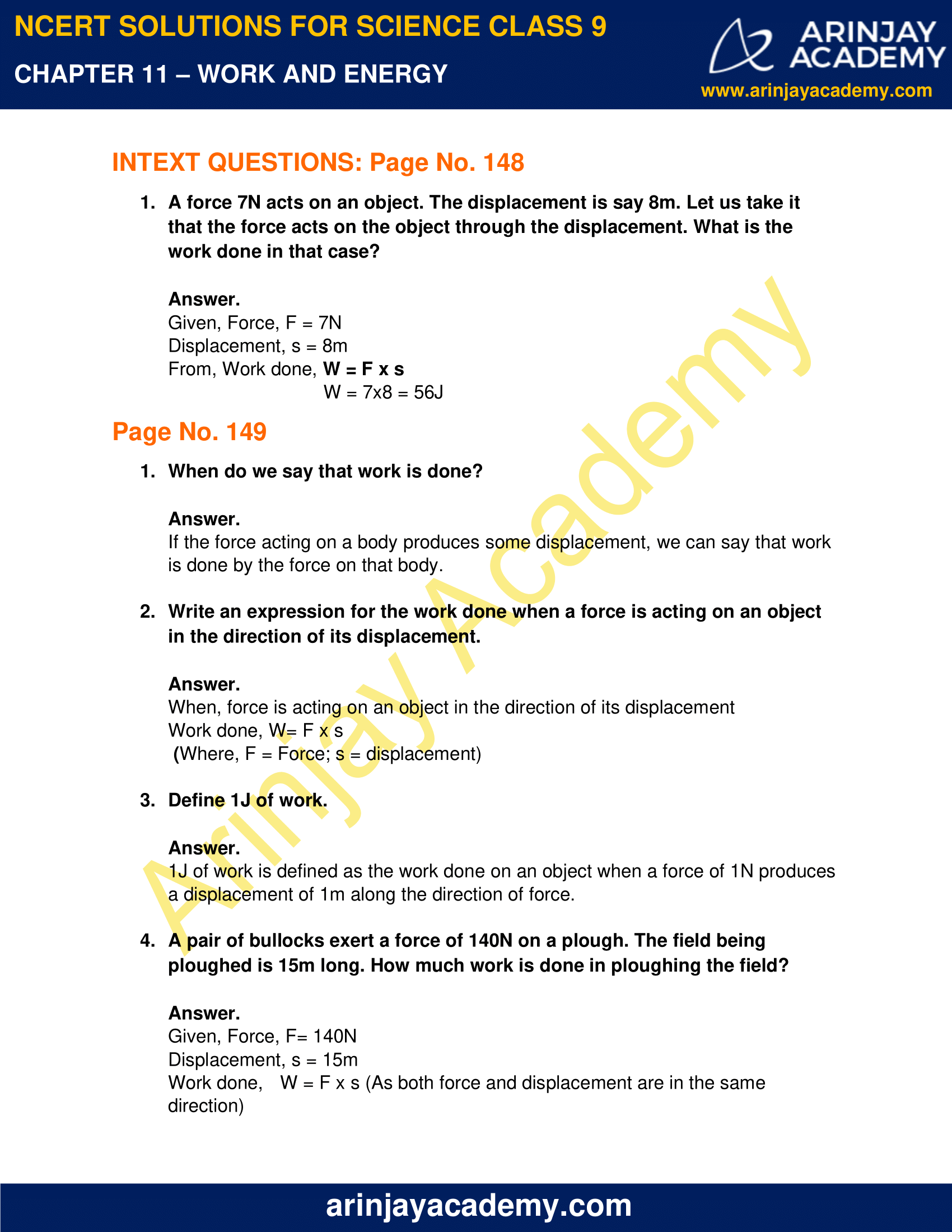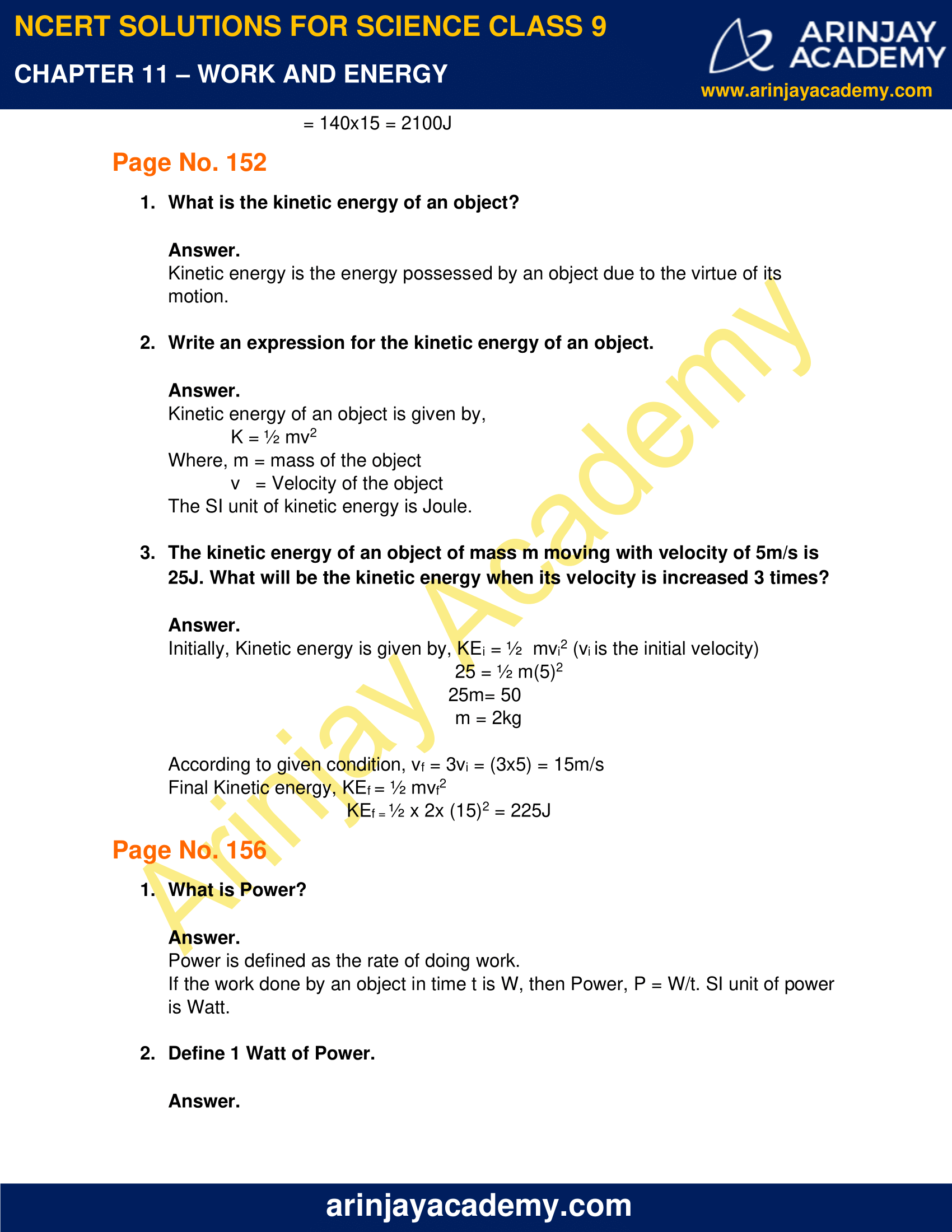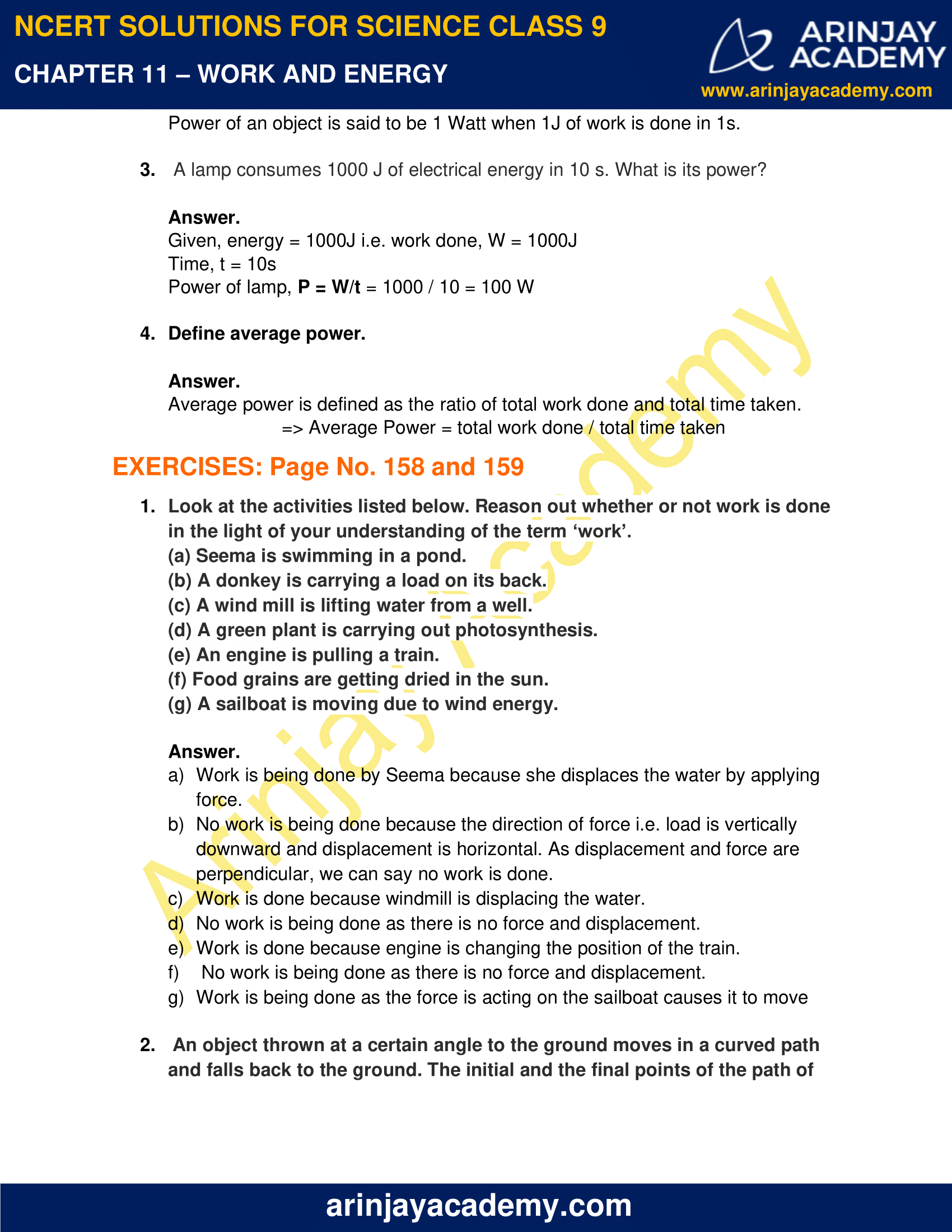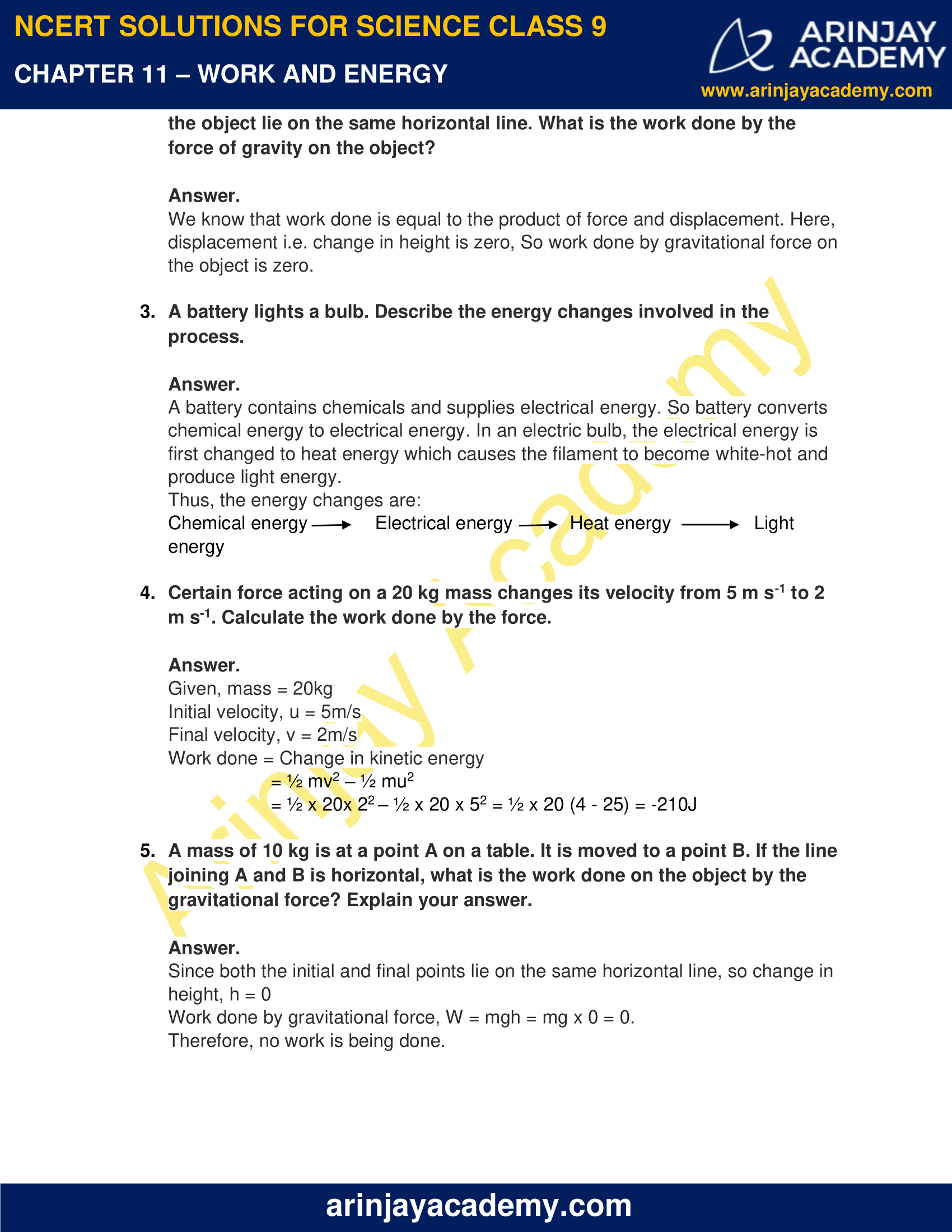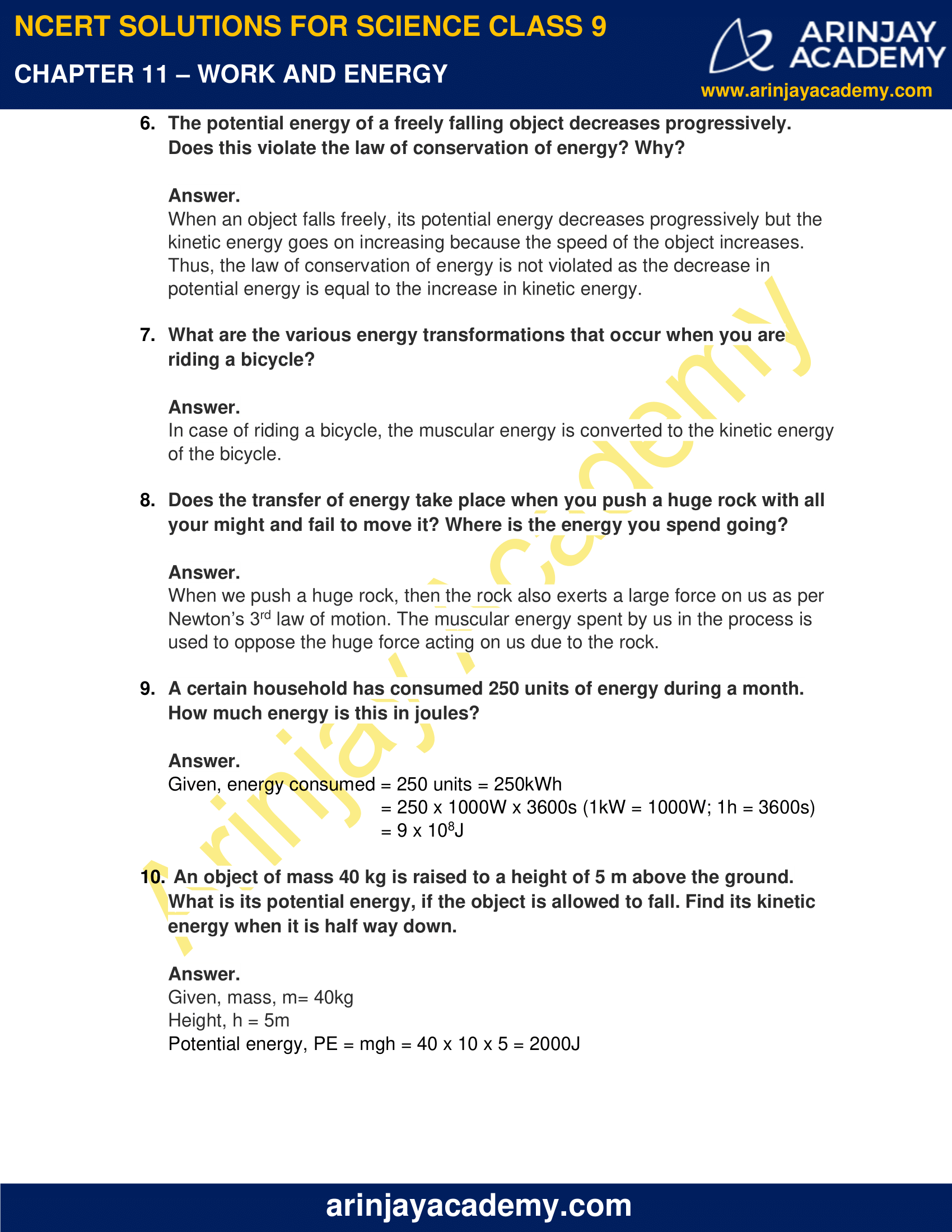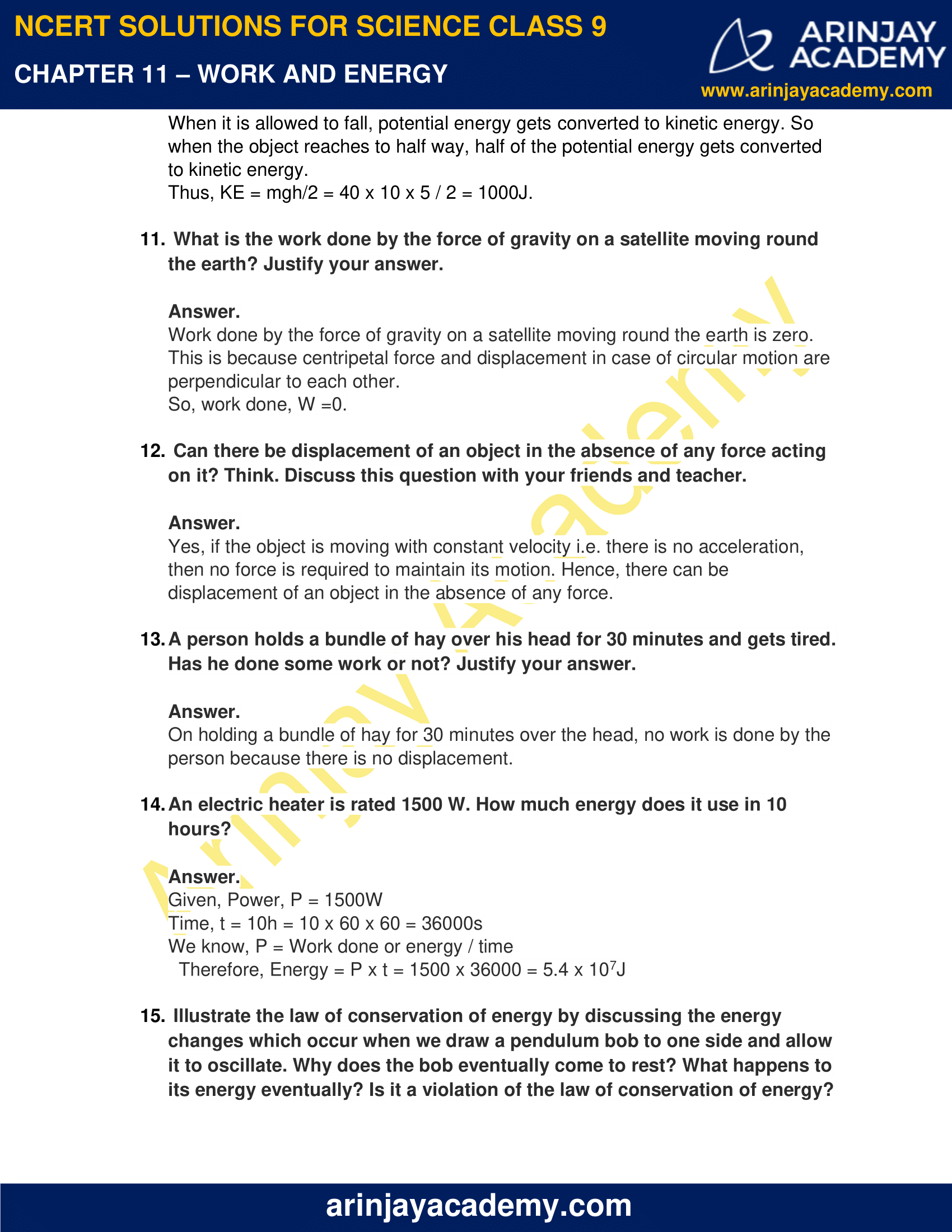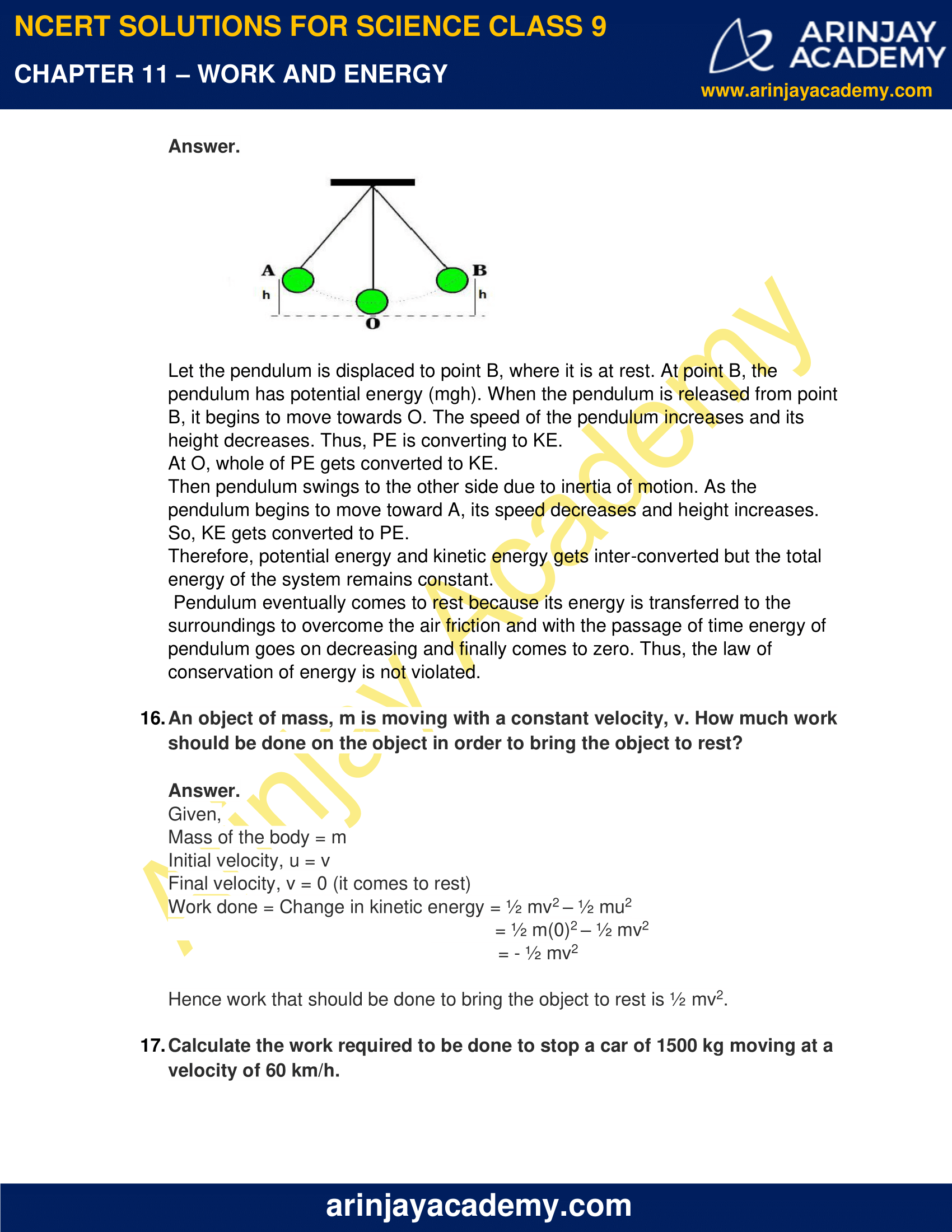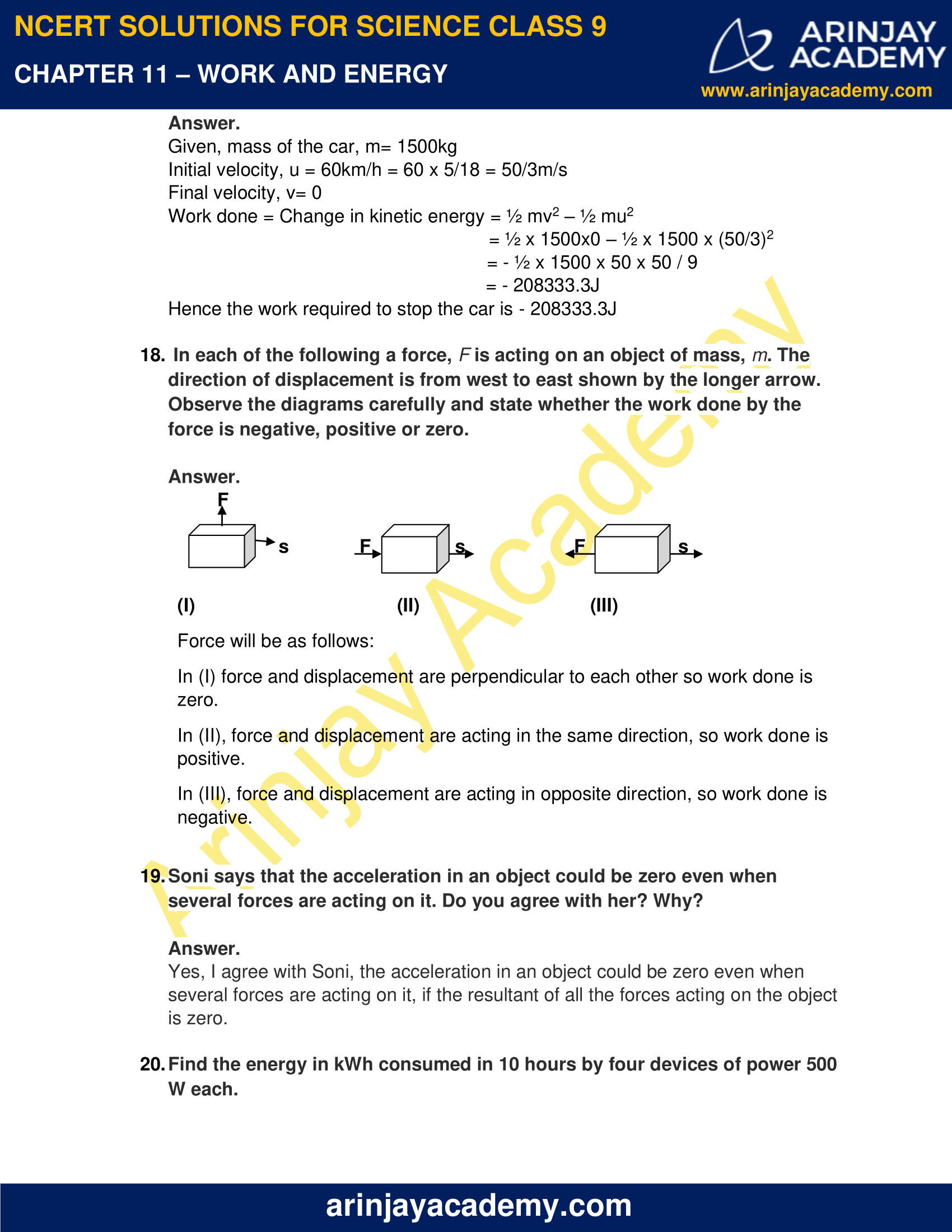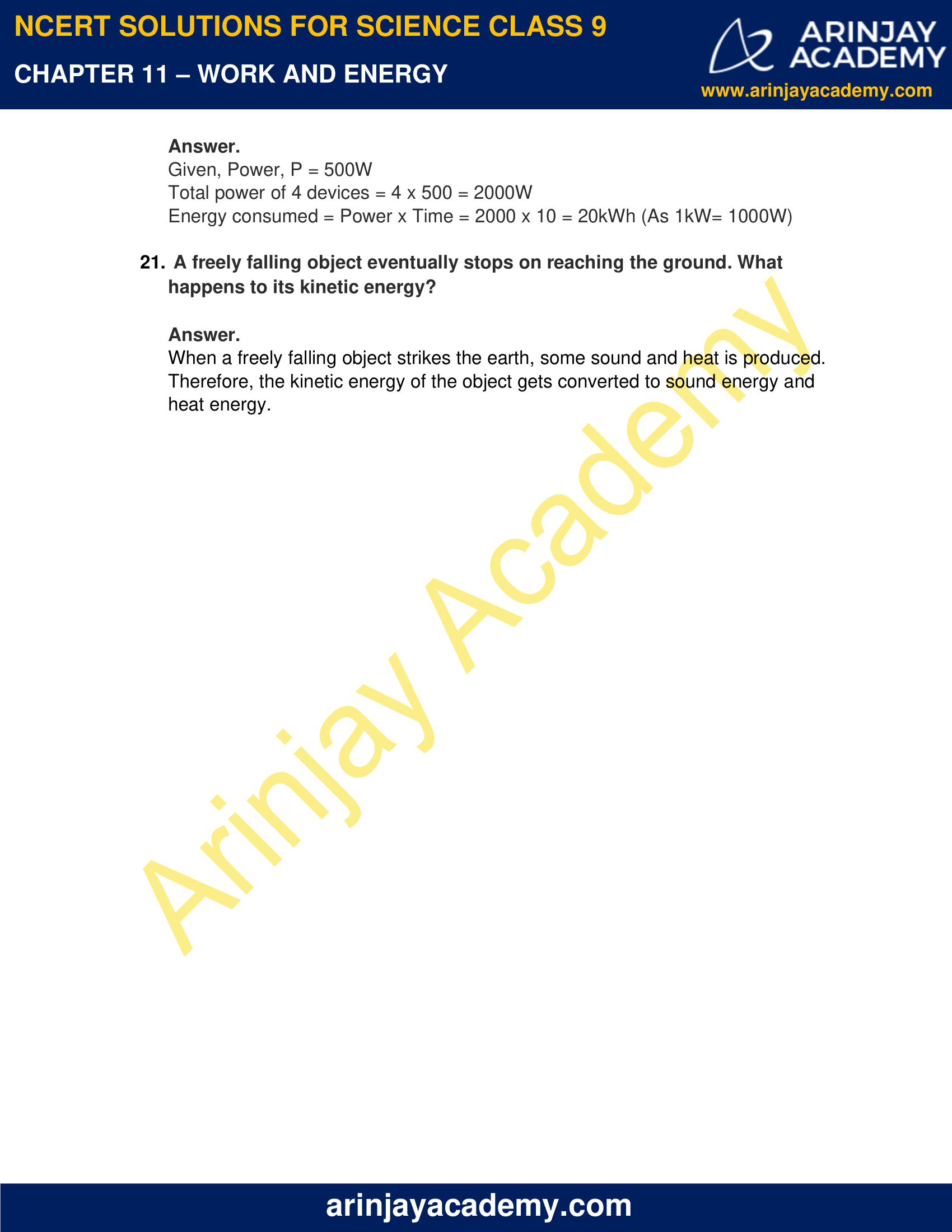### NCERT Solutions for Class 9 Science Chapter 11 – Work and Energy – NCERT Exercises

NCERT Solutions for Class 9 Science Chapter 11 – Work and Energy, Exercises includes all in text and exercise solved questions which helps you to understand the topic covered in Chapter 11 Work and Energy, in a better manner to help you to score good marks in your examinations.

### NCERT Solutions for Class 9 Science Chapter 11 – Work and Energy – Intext Questions: Page No. 148

1. A force 7N acts on an object. The displacement is say 8m. Let us take it that the force acts on the object through the displacement. What is the work done in that case?

Given, Force, F = 7N

Displacement, s = 8m

From, Work done, W = F x s

W = 7×8 = 56J

### Page No. 149

1. When do we say that work is done?

If the force acting on a body produces some displacement, we can say that work is done by the force on that body.

2. Write an expression for the work done when a force is acting on an object in the direction of its displacement.

When, force is acting on an object in the direction of its displacement

Work done, W= F x s

(Where, F = Force; s = displacement)

3. Define 1J of work.

1J of work is defined as the work done on an object when a force of 1N produces a displacement of 1m along the direction of force.

4. A pair of bullocks exert a force of 140N on a plough. The field being ploughed is 15m long. How much work is done in ploughing the field?

Given, Force, F= 140N

Displacement, s = 15m

Work done,   W = F x s (As both force and displacement are in the same direction)

= 140×15 = 2100J

### NCERT Solutions for Class 9 Science Chapter 11 – Work and Energy – Intext Questions – Page No. 152

1. What is the kinetic energy of an object?

Kinetic energy is the energy possessed by an object due to the virtue of its motion.

2. Write an expression for the kinetic energy of an object.

Kinetic energy of an object is given by,

K = ½ mv2

Where, m = mass of the object

v = Velocity of the object

The SI unit of kinetic energy is Joule.

3. The kinetic energy of an object of mass m moving with velocity of 5m/s is 25J. What will be the kinetic energy when its velocity is increased 3 times?

Initially, Kinetic energy is given by, KEi = ½  mvi2 (vi is the initial velocity)

25 = ½ m(5)2

25m= 50

m = 2kg

According to given condition, vf = 3vi = (3×5) = 15m/s

Final Kinetic energy, KEf = ½ mvf2

KEf = ½ x 2x (15)2 = 225J

### NCERT Solutions for Class 9 Science Chapter 11 – Work and Energy – Intext Questions – Page No. 156

1. What is Power?

Power is defined as the rate of doing work.

If the work done by an object in time t is W, then Power, P = W/t. SI unit of power is Watt.

2. Define 1 Watt of Power.

Power of an object is said to be 1 Watt when 1J of work is done in 1s.

3. A lamp consumes 1000 J of electrical energy in 10 s. What is its power?

Given, energy = 1000J i.e. work done, W = 1000J

Time, t = 10s

Power of lamp, P = W/t = 1000 / 10 = 100 W

4. Define average power.

Average power is defined as the ratio of total work done and total time taken.

=> Average Power = total work done / total time taken

### NCERT Solutions for Class 9 Science Chapter 11 – Work and Energy – NCERT Exercise – Page No. 158 and 159

1. Look at the activities listed below. Reason out whether or not work is done in the light of your understanding of the term ‘work’.
(a) Seema is swimming in a pond.
(b) A donkey is carrying a load on its back.
(c) A wind mill is lifting water from a well.
(d) A green plant is carrying out photosynthesis.
(e) An engine is pulling a train.
(f) Food grains are getting dried in the sun.
(g) A sailboat is moving due to wind energy.

a) Work is being done by Seema because she displaces the water by applying force.
b) No work is being done because the direction of force i.e. load is vertically downward and displacement is horizontal. As displacement and force are perpendicular, we can say no work is done.
c) Work is done because windmill is displacing the water.
d) No work is being done as there is no force and displacement.
e) Work is done because engine is changing the position of the train.
f) No work is being done as there is no force and displacement.
g) Work is being done as the force is acting on the sailboat causes it to move

2. An object thrown at a certain angle to the ground moves in a curved path and falls back to the ground. The initial and the final points of the path of the object lie on the same horizontal line. What is the work done by the force of gravity on the object?

We know that work done is equal to the product of force and displacement. Here, displacement i.e. change in height is zero, So work done by gravitational force on the object is zero.

3. A battery lights a bulb. Describe the energy changes involved in the process.

A battery contains chemicals and supplies electrical energy. So battery converts chemical energy to electrical energy. In an electric bulb, the electrical energy is first changed to heat energy which causes the filament to become white-hot and produce light energy.

Thus, the energy changes are: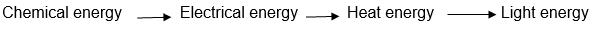4. Certain force acting on a 20 kg mass changes its velocity from 5 m s-1to 2 m s-1. Calculate the work done by the force.

Given, mass = 20kg

Initial velocity, u = 5m/s

Final velocity, v = 2m/s

Work done = Change in kinetic energy

= ½ mv2 – ½ mu2

= ½ x 20x 22 – ½ x 20 x 52 = ½ x 20 (4 – 25) = -210J

5. A mass of 10 kg is at a point A on a table. It is moved to a point B. If the line joining A and B is horizontal, what is the work done on the object by the gravitational force? Explain your answer.

Since both the initial and final points lie on the same horizontal line, so change in height, h = 0

Work done by gravitational force, W = mgh = mg x 0 = 0.

Therefore, no work is being done.

6. The potential energy of a freely falling object decreases progressively. Does this violate the law of conservation of energy? Why?

When an object falls freely, its potential energy decreases progressively but the kinetic energy goes on increasing because the speed of the object increases. Thus, the law of conservation of energy is not violated as the decrease in potential energy is equal to the increase in kinetic energy.

7. What are the various energy transformations that occur when you are riding a bicycle?

In case of riding a bicycle, the muscular energy is converted to the kinetic energy of the bicycle.

8. Does the transfer of energy take place when you push a huge rock with all your might and fail to move it? Where is the energy you spend going?

When we push a huge rock, then the rock also exerts a large force on us as per Newton’s 3rd law of motion. The muscular energy spent by us in the process is used to oppose the huge force acting on us due to the rock.

9. A certain household has consumed 250 units of energy during a month. How much energy is this in joules?

Given, energy consumed = 250 units = 250kWh

= 250 x 1000W x 3600s (1kW = 1000W; 1h = 3600s)

= 9 x 108J

10. An object of mass 40 kg is raised to a height of 5 m above the ground. What is its potential energy, if the object is allowed to fall. Find its kinetic energy when it is half way down.

Given, mass, m= 40kg

Height, h = 5m

Potential energy, PE = mgh = 40 x 10 x 5 = 2000J

When it is allowed to fall, potential energy gets converted to kinetic energy. So when the object reaches to half way, half of the potential energy gets converted to kinetic energy.

Thus, KE = mgh/2 = 40 x 10 x 5 / 2 = 1000J.

11. What is the work done by the force of gravity on a satellite moving round the earth? Justify your answer.

Work done by the force of gravity on a satellite moving round the earth is zero. This is because centripetal force and displacement in case of circular motion are perpendicular to each other.

So, work done, W =0.

12. Can there be displacement of an object in the absence of any force acting on it? Think. Discuss this question with your friends and teacher.

Yes, if the object is moving with constant velocity i.e. there is no acceleration, then no force is required to maintain its motion. Hence, there can be displacement of an object in the absence of any force.

13. A person holds a bundle of hay over his head for 30 minutes and gets tired. Has he done some work or not? Justify your answer.

On holding a bundle of hay for 30 minutes over the head, no work is done by the person because there is no displacement.

14. An electric heater is rated 1500 W. How much energy does it use in 10 hours?

Given, Power, P = 1500W

Time, t = 10h = 10 x 60 x 60 = 36000s

We know, P = Work done or energy / time

Therefore, Energy = P x t = 1500 x 36000 = 5.4 x 107J

15. Illustrate the law of conservation of energy by discussing the energy changes which occur when we draw a pendulum bob to one side and allow it to oscillate. Why does the bob eventually come to rest? What happens to its energy eventually? Is it a violation of the law of conservation of energy?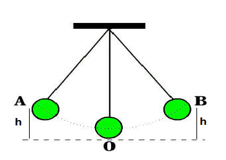Let the pendulum is displaced to point B, where it is at rest. At point B, the pendulum has potential energy (mgh). When the pendulum is released from point B, it begins to move towards O. The speed of the pendulum increases and its height decreases. Thus, PE is converting to KE.

At O, whole of PE gets converted to KE.

Then pendulum swings to the other side due to inertia of motion. As the pendulum begins to move toward A, its speed decreases and height increases. So, KE gets converted to PE.

Therefore, potential energy and kinetic energy gets inter-converted but the total energy of the system remains constant.

Pendulum eventually comes to rest because its energy is transferred to the surroundings to overcome the air friction and with the passage of time energy of pendulum goes on decreasing and finally comes to zero. Thus, the law of conservation of energy is not violated.

16. An object of mass, m is moving with a constant velocity, v. How much work should be done on the object in order to bring the object to rest?

Given,

Mass of the body = m

Initial velocity, u = v

Final velocity, v = 0 (it comes to rest)

Work done = Change in kinetic energy = ½ mv2 – ½ mu2

= ½ m(0)2 – ½ mv2

= – ½ mv2

Hence work that should be done to bring the object to rest is ½ mv2.

17. Calculate the work required to be done to stop a car of 1500 kg moving at a velocity of 60 km/h.

Given, mass of the car, m= 1500kg

Initial velocity, u = 60km/h = 60 x 5/18 = 50/3m/s

Final velocity, v= 0

Work done = Change in kinetic energy = ½ mv2 – ½ mu2

= ½ x 1500×0 – ½ x 1500 x (50/3)2

= – ½ x 1500 x 50 x 50 / 9

= – 208333.3J

Hence the work required to stop the car is – 208333.3J

18. In each of the following a force, is acting on an object of mass, m. The direction of displacement is from west to east shown by the longer arrow. Observe the diagrams carefully and state whether the work done by the force is negative, positive or zero.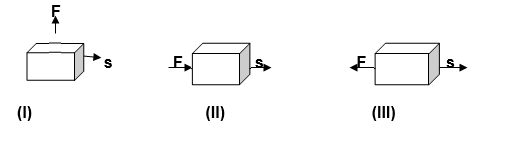Force will be as follows:

In (I) force and displacement are perpendicular to each other so work done is zero.

In (II), force and displacement are acting in the same direction, so work done is positive.

In (III), force and displacement are acting in opposite direction, so work done is negative.

19. Soni says that the acceleration in an object could be zero even when several forces are acting on it. Do you agree with her? Why?

Yes, I agree with Soni, the acceleration in an object could be zero even when several forces are acting on it, if the resultant of all the forces acting on the object is zero.

20. Find the energy in kWh consumed in 10 hours by four devices of power 500 W each.

Given, Power, P = 500W

Total power of 4 devices = 4 x 500 = 2000W

Energy consumed = Power x Time = 2000 x 10 = 20kWh (As 1kW= 1000W)

21. A freely falling object eventually stops on reaching the ground. What happens to its kinetic energy?